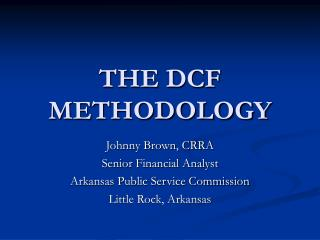DownloadDownload PresentationTHE DCF METHODOLOGY

# THE DCF METHODOLOGY

Download Presentation## THE DCF METHODOLOGY

- - - - - - - - - - - - - - - - - - - - - - - - - - - E N D - - - - - - - - - - - - - - - - - - - - - - - - - - -
##### Presentation Transcript

1. THE DCF METHODOLOGY Johnny Brown, CRRA Senior Financial Analyst Arkansas Public Service Commission Little Rock, Arkansas

2. Outline • What is the DCF? • Strengths & Weaknesses • How do I use the model? • New Twists

3. What is the DCF? • The model came about as the Dividend Discount Model. • P0 = D1/(1+k)1+…Dn/(1+k)n • Myron Gordon developed the model we know as the DCF Model. • k = D1/P0 + g

4. Strengths of the DCF • Easy to understand and use • Company specific information • Data required is readily available • Most wide-spread regulatory acceptance • Recognizes the time value of money and is forward-looking

5. Weaknesses of the DCF • Assumptions – don’t generally hold up in a technical sense • Growth rate to use is uncertain • Analyst growth forecasts are short-term/DCF is long-term • Sometimes difficult to match growth with the yield component • Efficient Market Hypothesis is not universally accepted

6. Putting the Model Together • k = D1/P0 + g • The analyst must provide the components on the right side of the equation to solve for “k”. • Match the right side with investor’s expectations. • Each component is highly scrutinized by other witnesses. • The result is an accurate measurement of the cost of equity.

7. Derivation of “D” • The dividend component is probably the least debated part of the DCF equation. • The dividend should not be influenced by short-term anomalies. • D1 = Annualized dividend at time period 1 or • D1= quarterly dividend multiplied by four (D0) and grossed up by the annual growth rate, “g”

8. Derivation of “D” • Example: Quarterly dividend = \$.50 D0 would equal \$2.00 (.50 x 4 = 2) D1 would equal \$2.10 (2.00 x (1+g); g=5%)

9. Derivation of “P” • The price should also not be influenced by short-term anomalies. • The price should be taken from the same time period as the dividend and growth data. • Doing so should account for investors’ perception of the company’s risk and return prospects.

10. Derivation of “P” • I like to use an average of a recent time period – average of 13 weekly price points • 13 weeks = 1 quarter • Most analyst growth projections are published quarterly.

11. Derivation of “g” • The most CONTROVERSIAL part of the DCF

12. Derivation of “g” • Forward looking – but influenced by historical growth information • Utility industry is mature and slow growing • Remember – you are measuring long-term sustainable growth in dividends.

13. DCF Method • Example: Analyst derived information: • Quarterly Div. - \$0.25 • 13 week average price – \$25 • Annual growth rate – 5%

14. DCF Method • k = D1/P0 + g k = 1.05/25 + .05 k = 9.2%

15. New Twists • Title of panel is New Twists on DCF – my presentation doesn’t jibe. • That’s my point – if it ain’t broke don’t fix it.

16. Market to Book Value Adjustment • Not necessary – allows for over recovery • For a regulated utility – a market price above one indicates investors expect returns above what is required. • Why else would they be willing to pay above book value for their investment? • The fact that most regulators only allow utilities a return based on book value rate base is widely known by investors.

17. Growth in Dividends is the key! Johnny Brown Arkansas Public Service Commission 501-682-5743 johnny_brown@psc.state.ar.us